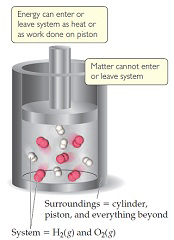# Problem: You may want to reference (Pages 172 - 176)Section 5.3 while completing this problem.A gas is confined to a cylinder under constant atmospheric pressure, as illustrated in the following figure. When 0.460 kJ of heat is added to the gas, it expands and does 216 J of work on the surroundings.What is the value of ΔH for this process?

###### FREE Expert Solution

At constant pressure, q = ΔH:

$\mathbf{∆}\mathbf{H}\mathbf{=}\mathbf{+}\mathbf{q}$

• q → (+) → heat is added
94% (19 ratings)###### Problem Details

You may want to reference (Pages 172 - 176)Section 5.3 while completing this problem.

A gas is confined to a cylinder under constant atmospheric pressure, as illustrated in the following figure. When 0.460 kJ of heat is added to the gas, it expands and does 216 J of work on the surroundings.What is the value of ΔH for this process?

Frequently Asked Questions

What scientific concept do you need to know in order to solve this problem?

Our tutors have indicated that to solve this problem you will need to apply the Internal Energy concept. You can view video lessons to learn Internal Energy. Or if you need more Internal Energy practice, you can also practice Internal Energy practice problems.